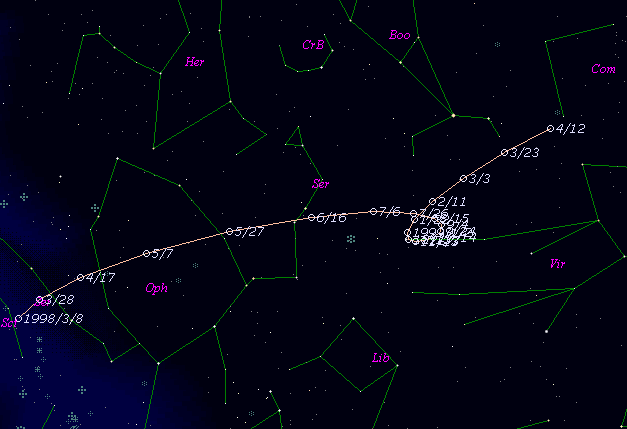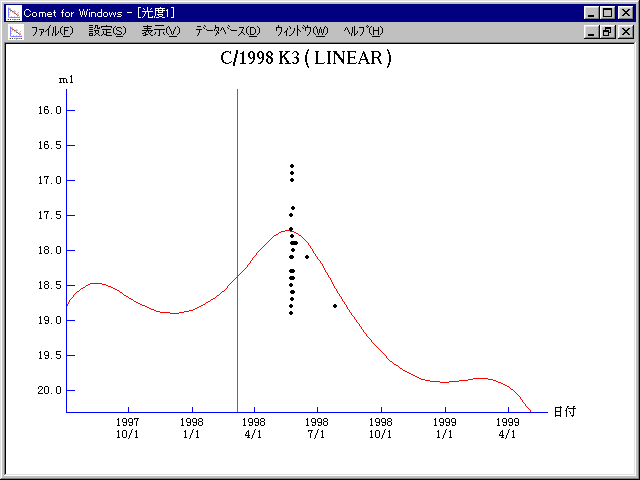# \$B%j%K%"WB@1(B

C/1998 K3 ( LINEAR )###\$B%W%m%U%#!<%k(B

 \$BH/8+F|(B 1998\$BG/(B5\$B7n(B23\$BF|(B \$BH/8+8wEY(B 18.4\$BEy(B \$BH/8+ Lincoln Laboratory Near-Earth Asteroid Research project

###\$B50F;MWAG(B

```   The following improved orbital elements, by Kenji Muraoka are
from 171 observations  1998 May 23  to  July 26, perturbations
by 9 Planets, Moon and 5 minor planets were taken into account.
The mean residual is +/- 0.64 arc seconds.

Epoch  =  1998 Mar.  8.0  TT       JDT = 2450880.5
T  =  1998 Mar.  7.16519       +/- 0.01261 (m.e.) TT
Peri. =   47.82312                +/- 0.00301
Node  =  307.94592                +/- 0.00250   (2000.0)
Incl. =  160.20549                +/- 0.00111
q  =    3.5462549              +/- 0.0000979 AU
e  =    0.9974469              +/- 0.0005278
1/a  =   +0.0007199              +/- 0.0001488 1/AU
( P  =  51768 years )
```

###\$B@1?^(B###\$B8wEYJQ2=(B

```        m1 = 10.0 + 5 log\$B&\$(B + 10.0 log r
```##### \$B50F;MWAG\$OB<2,7r<#;a\$N7W;;\$K\$h\$k\$b\$N\$G\$9!#(B \$B@1?^\$O(B StellaNavigator Ver.2.0 for Windows (\$B%"%9%H%m%"!<%D(B \$BJTCx(B / \$B%"%9%-!<=PHG6I4)(B) \$B\$G:n@.\$7\$?\$b\$N\$G\$9!#(B \$B8wEY%0%i%U\$O(BComet for Windows\$B\$G:n@.\$7\$?\$b\$N\$G\$9!#(B